Download Presentation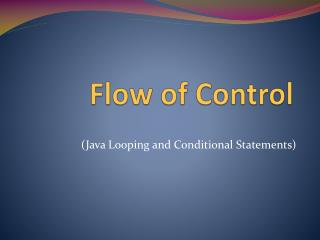Flow Control

# Flow Control - PowerPoint PPT Presentation

astig! m/I am the owner, or an agent authorized to act on behalf of the owner, of the copyrighted work described.
Download Presentation## Flow Control

An Image/Link below is provided (as is) to download presentation

Download Policy: Content on the Website is provided to you AS IS for your information and personal use and may not be sold / licensed / shared on other websites without getting consent from its author.While downloading, if for some reason you are not able to download a presentation, the publisher may have deleted the file from their server.

- - - - - - - - - - - - - - - - - - - - - - - - - - E N D - - - - - - - - - - - - - - - - - - - - - - - - - -
Presentation Transcript### Flow of Control

(Java Looping and Conditional Statements)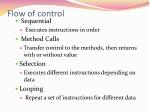Flow of control
•  Sequential
•  Executes instructions in order
•  Method Calls
• Transfer control to the methods, then returns with or without value
• Selection
• Executes different instructions depending on data
• Looping
•  Repeat a set of instructions for different data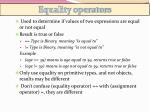Equality operators

•  Used to determine if values of two expressions are equal or not equal
• Result is true or false
•  == Type is Binary, meaning “is equal to”
•  != Type is Binary, meaning “is not equal to”

Example

•  (age == 39) means is age equal to 39, returns true or false
•  (age != 39) means is age not equal to 39, returns true or false
• Only use equality on primitive types, and not objects, results may be different
•  Don’t confuse (equality operator) == with (assignment operator) =, they are different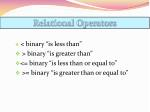Relational Operators

• < binary “is less than”
• > binary “is greater than”
• <= binary “is less than or equal to”
• >= binary “is greater than or equal to”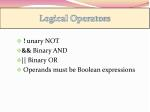Logical Operators

• ! unary NOT
• && Binary AND
• || Binary OR
•  Operands must be Boolean expressions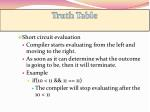Truth Table

• Short circuit evaluation
• Compiler starts evaluating from the left and moving to the right.
• As soon as it can determine what the outcome is going to be, then it will terminate.
• Example
•  if(10 < 11 && 11 == 11)
• The compiler will stop evaluating after the 10 < 11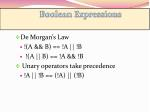Boolean Expressions

• De Morgan’s Law
• !(A && B) == !A || !B
•  !(A || B) == !A && !B
•  Unary operators take precedence
• !A || !B == (!A) || (!B)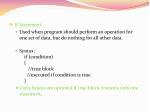If Statement

• Used when program should perform an operation for one set of data, but do nothing for all other data.
• Syntax:    if (condition)    {       //true block      //executed if condition is true    }
• Curly braces are optional if true block contains only one statement.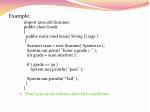Example:

import java.util.Scanner;public class Grade{  public static void main( String [] args ) {   Scanner scan = new Scanner( System.in );   System.out.print( "Enter a grade > " );   int grade = scan.nextInt( );   if ( grade >= 50 )      System.out.println( "pass" );   System.out.println( "Fail" );  }}

• Don’t put semi-colons after the conditionIf Else Statement

•  Used when program should perform an operation when a condition is true for one set of data, and then do something else when the condition is false another set of data
•  Again, no need for curly braces if only one statement within conditionExample:

import java.util.Scanner;public class Divid{   public static void main( String [] args )  {    Scanner scan = new Scanner( System.in );    System.out.print( "Enter the dividend > " );    int dividend = scan.nextInt( );   System.out.print( "Enter divisor > " );   int divisor = scan.nextInt( );   if ( divisor == 0 )      System.out.println( "divide by zero" );  else    System.out.println( dividend + " / " + divisor   + " = " + ( dividend / divisor ) );  }}If Else If Statement

• Used when program should perform an operation when a condition is true for one set of data, and then evaluate another operation when the first condition is false.
• Nested if statements
• Used when program should evaluate another condition when a condition evaluates to trueExamples of terminating nested if statements

• Dangling Else
• Generates compiler error
• Testing techniques
• A good test suite executes every statement in your program (could be exhaustive).
• Comparing doubles/floats decimals

Give yourself a thresholdif(Math.abs(dbl1 – dbl2) < .0001) …Comparing characters

• Comparisons of characters are comparing the Unicode number of the character
• Comparing objects
• Comparisons of objects that do not override the Object.equals method are comparing the instance of object in memory, not the data of the object
• compare objects use the equals method.Comparing strings

• Strings are objects, so use the equals method
• You can also use equalsIgnoreCase are defined in the string class and compareTo – used for sorting
• Conditional operator
• A shortcut to simplify a simple if else statement
• ExampleString result = (testString == “help” ? “needs help” : “does not need help”);Switch Statement

• Only accepts character or integer (byte, short, or int) expression to constants of the same type.

Can group case statementscase 1:case 2:// do somethingbreak;### Arrays

Arrays are to data as loops are to control flow.Arrays
• Arrays are the basic way to store large numbers of values. Every programming language you're likely to use has arrays as part of the fundamental language, altho with many little variations on how they work.An array can store many similar values in memory. Each value is accessed by specifying an integer subscript or index in brackets following the array name. "Array" in Java means approximately the same thing as array, matrix, or vector does in math. Unlike math and some other programming languages, in Java you must both declare an array and allocate a fixed amount of memory for it.Declaring an Array
• An array variable is like other variables -- you must declare it, which means you must declare the type of elements that are in an array. All elements must be the same type. Write the element type name, then "[]", then the name of the array variable.The declaration allocates only enough space for a reference to an array (typically 4 bytes), but doesn't create the actual array object.

• String[] args; // args is an array of Strings
• int[] scores; // scores is an array of ints
• JButton[] controlButtons; // controlButtons is an array of JButtons
• No size in declaration. Unlike some languages, never put the size of the array in the declaration because an array declaration specifies only the element type and the variable name. The size is specified when you allocate space for the array.• Most programming guidelines suggest using plural names, or nouns denoting a collection of things, for arrays and other collections of multiple values. This is not a rigid rule, and your choice will often be based on linguistic sensitives.Allocate an array object with new
• Create an array using new. This example creates an array of 100 int elements, from a to a.

int[] a; // Declare a to be an array of ints

a = new int; // Allocate an array of 100 ints

These are often combined in one line.

int[] a = new int; // Declare and allocate.Subscripts (indexes/indices)
• Subscripts are enclosed in square brackets []. xi in mathematics is x[i] in Java, and is pronounced "x-sub-i".
• Subscript ranges always start at zero because Java came largely from C++, which had a good reason for using zero (pointer arithmetic on arrays).Java always checks subscript legality to be sure the subscript is >= 0, and less than the number of elements in the array. If the subscript is outside this range, Java throws ArrayIndexOutOfBoundsException. This is far superior to the behaver of C and C++, which allow out of range references. Consequently, Java programs are far less susceptible to bugs and security flaws than C/C++ programs.Zero-based indexing
• is a constant annoyance of Java's zero-based indexing. The natural human value for hours or days should be used as an index to make the program most readable, but this would mean starting with 1, not zeroInitial array element values -- zero/null/false
• When an array is allocated (with new), all elements are set to an initial value. The initial value is 0 if the element type is numeric (int, float, ...), false for boolean, and null for all object typesArray Initialization
• When you declare an array, you can can also allocate a preinitialized array object in the same statement. In this case, do not give the array size because Java counts the number of values to determine the size.

For example:

String[] days = {"Su", "Mo", "Tu", "We", "Th", "Fr", "Sa"};Array diagrams
• This code declares and initializes an array.

int[] a = {1, 2, 4, 8, 16};

Arrays are often represented with diagrams that represent their memory use.Each box represents the amount of memory needed to hold one array element. For ints this is 4 bytes. The array variable, a, is a reference (ie, memory address in the heap) of the block of memory that holds the actual array elements.References are often represented by a black disk with an arrow pointing to the data they reference. Most often array diagrams are written vertically, but sometimes the cells are arranged horizontally, especially for arrays of characters. Of course in the actual physical memory there is no such idea as vertical or horizontal.Array variables are references to a block of elements
• When you declare an array variable, Java reserves only enough memory for a reference(Java's name for an address or pointer) to an array object. References typically require only 4 bytes. When an array object is created with new, a reference is returned, and that reference can then be assigned to a variable. When you assign one array variable to another, only the reference is copiedFor example:

int[] a = new int[] {100, 99, 98}; // "a" references the array object.

int[] b; // "b" doesn't reference anything.

b = a; // Now "b" refers to the SAME array as "a"

b = 0; // Also changes a because a and b refer to the same arrayCommon array problems

Some common array programming mistakes are:

• Runtime error: Forgetting that array subscripts start with zero.
• Compile-time error: Writing a.length() instead of a.length. The length() method is used with Strings, not arrays.
• Compile-time error: Declaring an array with a size. Eg, int a; instead of int[] a = new int;.